## Centroids

Conclusion:The state of mind of the observer plays a crucial role in the perception of time.Einstein

It is often I consider the Center of Gravity(Mass) as an illustration of the perspective that exists “around and within us” as a product of the natural world. We are limited by our own knowledge, yet when given, and lead to observation of what is natural in our world, we see where we had not seen before.

\bar z = \frac{\int z dm}{m}The center of gravity (or mass), abbreviated as COM, of any object is that point within the object upon which gravity (or any body force) acts, regardless of the orientation of the object. The COM of an object may be calculated by using the principle of equilibrium.

First off you must know that I work according to a set of principles that were discovered after a time through “subjective reflection” on the nature of mind and how it works. Of course I am no expert here, but the principles themself are realizable as an active function in the recognition of how one might interpret the world.

\bar y = \frac{\int y \rho dV}{\int \rho dV}In the event that the density ρ of an object is not uniform throughout, the calculation of COM may be done by a similar set of equations involving the addition of density to the analysis.

It is by taking one to recognisance of the title of this blog entry that I wanted to discuss the “subjective part” and the understanding of the “mathematical construct” that one, in my opinion exists. Just as we progress to understand the natural world around us, I believe these are synonymous with each other.

\bar Y = \frac{\sum m \bar y}{\sum m}If a body is made up of multiple sections, each of which has a unique mass, the method for evaluating the centroid of that body is to evaluate the composite body by finite element analysis of each of the sections through the use of moment balancing, as above.

Liminocentric structuresYou can find much on this site in regards to this issue, and I now relate centroid for consideration in the case of this topic. I presented it at the Backreaction site today to show an advancement in the thinking as we move to incorporate the knowledge. As we come to understand the natural world around us.

Giving earth a new understanding in terms of its densities and it’s relation to the gravitational contribution, it has in our new views of the globe, helped me learn and understand the feature of gravity as it extends to the cosmos.

I have taken it a step further as many of you know to include the emotive states as valued physiological relations to what manifests in the immediate environs of the body home. While this is a far cry from the understanding of gravitational research it was inevitable for me to see an extension of how one can topological relate to the world, in this inductive/deductive mode to reason.

While living on this earth, we always come home for reflection, and if any have not taken stock of what has accumulated during the day, then it is inevitable that is will come to reside very close to home as one sleeps through the nocturnality night. This research of subjectivity and mind had to entail “all of our activities.” It had to include an understanding of the “mathematical constructs” that we observe, in order to relate to nature.

List of Centroids

.nobrtable br { display: none }

Shape Figure$\bar x$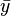$\bar y$ Area
Triangular area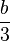$\frac{b}{3}$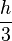$\frac{h}{3}$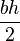$\frac{bh}{2}$
Quarter-circular area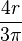$\frac{4r}{3\pi}$$\frac{4r}{3\pi}$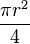$\frac{\pi r^2}{4}$
Semicircular area$\,\!0$$\frac{4r}{3\pi}$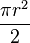$\frac{\pi r^2}{2}$
Quarter-elliptical area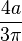$\frac{4a}{3\pi}$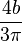$\frac{4b}{3\pi}$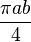$\frac{\pi a b}{4}$
Semielliptical area The area inside the ellipse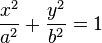$\frac{x^2}{a^2} + \frac{y^2}{b^2} = 1$ and above the$\,\!x$ axis$\,\!0$$\frac{4b}{3\pi}$$\frac{\pi a b}{2}$
Semiparabolic area The area between the curve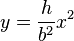$y = \frac{h}{b^2} x^2$ and the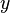$\,\!y$ axis, from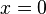$\,\!x = 0$ to$\,\!x = b$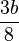$\frac{3b}{8}$$\frac{3h}{5}$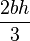$\frac{2bh}{3}$
Parabolic area The area between the curve$\,\!y = \frac{h}{b^2} x^2$ and the line$\,\!y = h$$\,\!0$$\frac{3h}{5}$$\frac{4bh}{3}$
Parabolic spandrel The area between the curve$\,\!y = \frac{h}{b^2} x^2$ and the$\,\!x$ axis, from$\,\!x = 0$ to$\,\!x = b$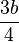$\frac{3b}{4}$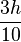$\frac{3h}{10}$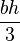$\frac{bh}{3}$
General spandrel The area between the curve$y = \frac{h}{b^n} x^n$ and the$\,\!x$ axis, from$\,\!x = 0$ to$\,\!x = b$$\frac{n + 1}{n + 2} b$$\frac{n + 1}{4n + 2} h$$\frac{bh}{n + 1}$
Circular sector The area between the curve (in polar coordinates)$\,\!r = \rho$ and the pole, from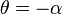$\,\!\theta = -\alpha$ to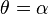$\,\!\theta = \alpha$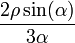$\frac{2\rho\sin(\alpha)}{3\alpha}$$\,\!0$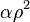$\,\!\alpha \rho^2$
Quarter-circular arc The points on the circle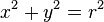$\,\!x^2 + y^2 = r^2$ and in the first quadrant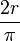$\frac{2r}{\pi}$$\frac{2r}{\pi}$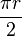$\frac{\pi r}{2}$
Semicircular arc The points on the circle$\,\!x^2 + y^2 = r^2$ and above the$\,\!x$ axis$\,\!0$$\frac{2r}{\pi}$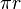$\,\!\pi r$
Arc of circle The points on the curve (in polar coordinates)$\,\!r = \rho$, from$\,\!\theta = -\alpha$ to$\,\!\theta = \alpha$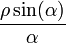$\frac{\rho\sin(\alpha)}{\alpha}$$\,\!0$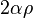$\,\!2\alpha \rho$

Why are Planets Round?

It is always interesting to see water in space.

Planets are round because their gravitational field acts as though it originates from the center of the body and pulls everything toward it. With its large body and internal heating from radioactive elements, a planet behaves like a fluid, and over long periods of time succumbs to the gravitational pull from its center of gravity. The only way to get all the mass as close to planet’s center of gravity as possible is to form a sphere. The technical name for this process is “isostatic adjustment.”

With much smaller bodies, such as the 20-kilometer asteroids we have seen in recent spacecraft images, the gravitational pull is too weak to overcome the asteroid’s mechanical strength. As a result, these bodies do not form spheres. Rather they maintain irregular, fragmentary shapes.

This entry was posted in Inverse Square Law, L5, lagrangian, Liminocentric. Bookmark the permalink.